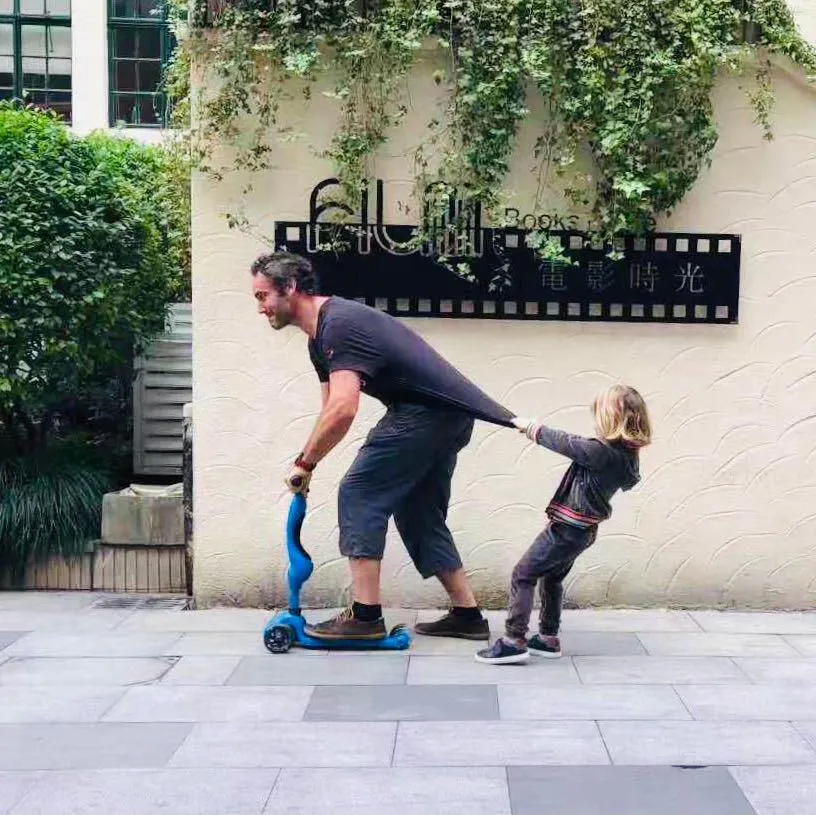My Daughter's Laptop

It's a programable "laptop." Hardware that allows me to program different activities in a nice box.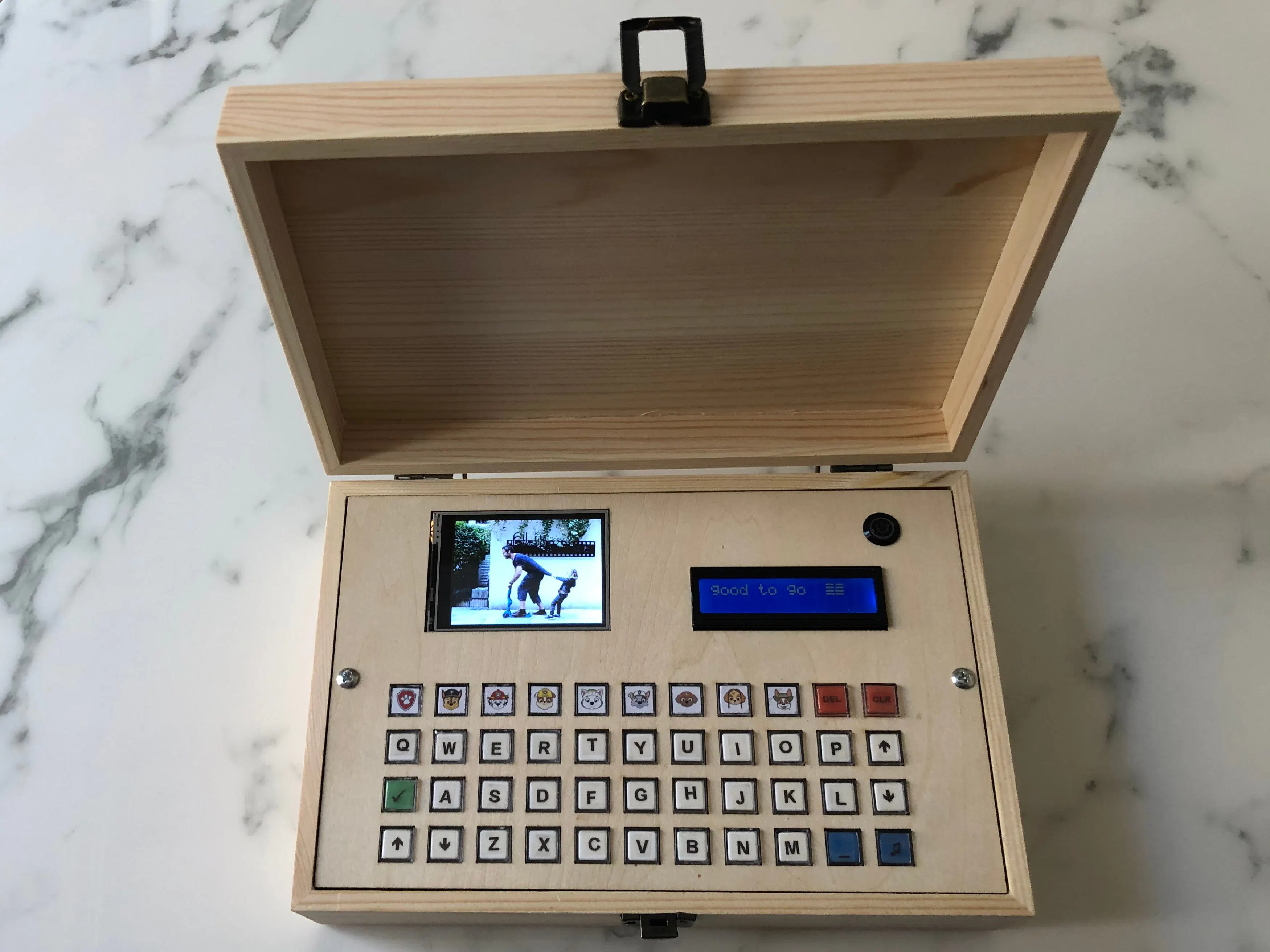Things used in this project

Hardware components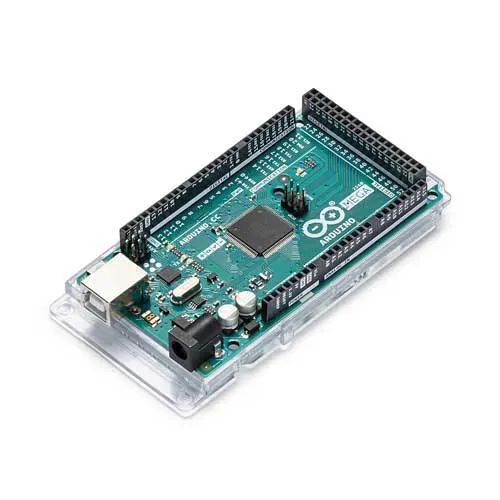Arduino Mega 2560
×1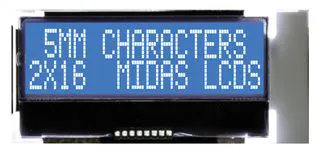Alphanumeric LCD, 16 x 2
×1
 ILI9341
×1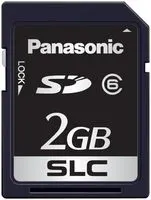Flash Memory Card, SD Card
×2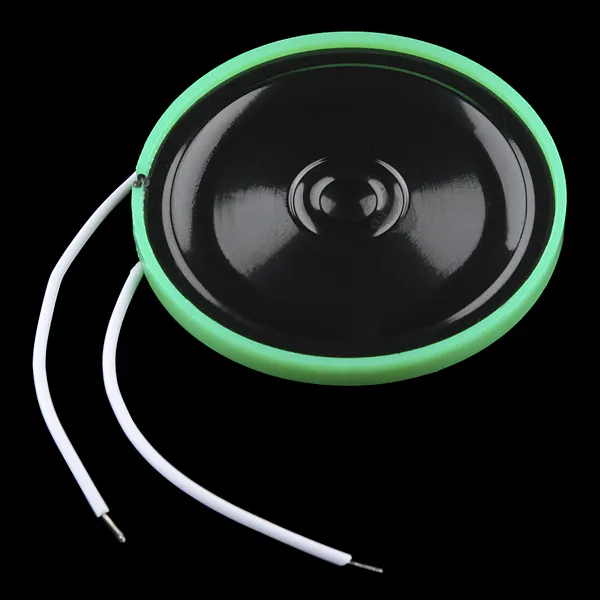Speaker: 0.25W, 8 ohms I use a 3W one
×1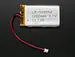Li-Ion Battery 1000mAh
×1
 DFPlayer - A Mini MP3 Player
×1
 3.7V 4.2V Charger & 5V 6V 9V 12V Discharger Board DC DC Converter Boost Module for UPS mobile power 18650 lithium battery
×1
 DC 5-12V NE555 Switch Module Relay Shield Timer 0-150 Second Adjustable L2KD
×1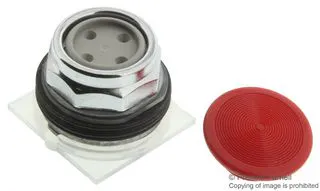Switch Actuator, Head for spring return push-button
×1

Schematics

Schematics of all project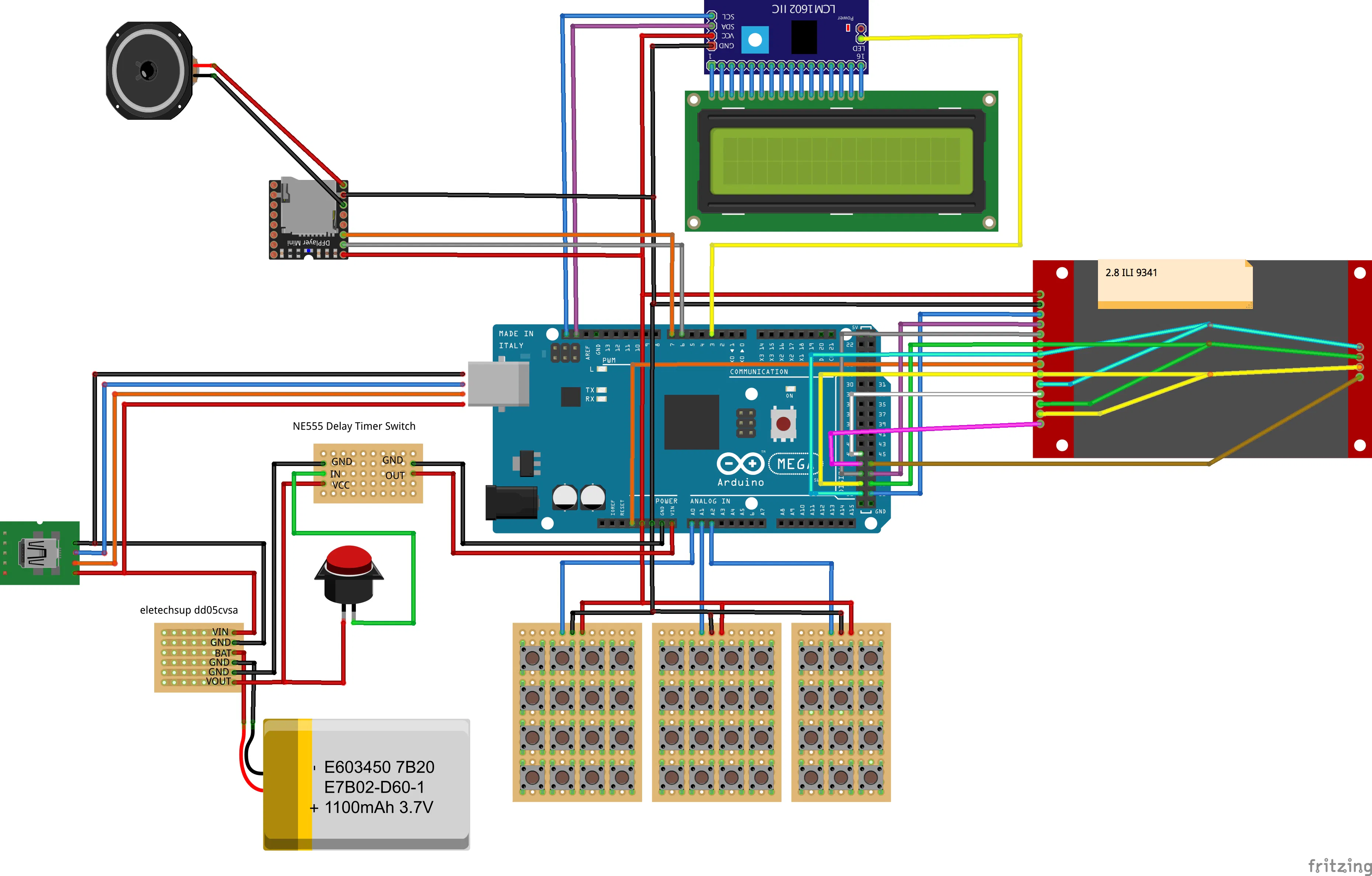Code

The code for the spelling game

Arduino
This game shows a picture on the ILI9341. On the keyboard, there are 10 drawings.
After pressing one of those keys a Picture of the animal appears on the screen, the name is written on the LCS 16x2, and a track with the sound plays on the mp3. Then after you type it on the keyboard if the name is correct it sounds music to celebrate .
//LCD
#include <ILI9341_kbv.h> // This library has been modified
ILI9341_kbv my_lcd;
#define vali0 0

//SD CARD
#include <SD.h>
#define TFT_SD 47  //SC_CS
Sd2Card card;
SdVolume volume;
SdFile root;

//MP3 Mini
#include "DFRobotDFPlayerMini.h"
//#include "Arduino.h"
#include "SoftwareSerial.h"
SoftwareSerial mySoftwareSerial(7,6); // RX, TX
DFRobotDFPlayerMini myDFPlayer;

//LCD 2 lines
#include <LiquidCrystal_I2C.h>// LCD  Library and variables
LiquidCrystal_I2C lcd(0x27,16,2);
int lcdLED = 3; // brignes of the LCD
int bright; // To store the screen brightness

//TFT Touch Library
#include <LCDWIKI_TOUCH.h>
LCDWIKI_TOUCH my_tft(44,52,50,51,46); //tcs,tclk (sharing with LCD),tdout (MISO) ,tdin (MOSI) ,tirq

//Folder on the SD card to keep the pictures
String FOLDERROOT = ""; // I have no folder because all the pictures are on the root of the SD
uint8_t max_img = 40;
uint8_t image_counter = 0;
unsigned long auxtimer, colortimer;

int analogPin0 = A0; // Or any pin you are using
int analogPin1 = A1; // Or any pin you are using
int analogPin2 = A2; // Or any pin you are using

//Aux Global variables
String song;
String dog;
String mydog;

void setup()
{
Serial.begin(9600);
//Initiate LCD big screen
my_lcd.begin();
my_lcd.setRotation(3);// Landscape
my_lcd.fillScreen(TFT_PINK);// set color iitial screen
//Initiate the Touch capabilities
SD.begin(TFT_SD);
my_tft.TP_Init(1,240,320);  //Define the values for my_lcd ( rotation,With,Height)

// initialize the lcd
Serial.print("\nInitializing LCD...");
bright =70;// brignes of the LCD
analogWrite(lcdLED, bright);// brignes of the LCD
lcd.init();
lcd.backlight();
lcd.setCursor(0,0);

//Inicialize mp3
Serial.print("\nInitializing MP3...");
mySoftwareSerial.begin(9600);
if (!myDFPlayer.begin(mySoftwareSerial)) //Use softwareSerial to communicate with mp3.
{
lcd.println("Unable to begin:");
// while(true); //wait until fixed
}
myDFPlayer.setTimeOut(500); //Set serial communictaion time out 500ms
myDFPlayer.volume(25);  //Set volume value (0~30).
myDFPlayer.EQ(DFPLAYER_EQ_NORMAL);
myDFPlayer.outputDevice(DFPLAYER_DEVICE_SD);

//All good

//Fist picture
Serial.print("\nInitializing BMP...");
String filename ="pp_1.bmp";
int len = 30;
char pepito[len];
filename.toCharArray(pepito, len);
bmpDraw(pepito , 0, 0);
lcd.init();
lcd.backlight();
lcd.setCursor(0,0);
myDFPlayer.play(28);
lcd.println("good to go  ");

}

// Makes an Array out of a BMP
void bmp (String filename){
int len = 30;
char pepito[len];
filename.toCharArray(pepito, len);
bmpDraw(pepito , 0, 0);}

String PAW (String dog)//PRINT THE NAME OF THE DOG
{
lcd.init();
lcd.setCursor(0,0);
lcd.print(dog);
MP3(dog);
delay(50);
mydog =""; //Zero mydog variable
return dog; // return the name of the dog to the main program
}

void TYPE( String key, String dog) // print the keyboar imput and compares to the dog name
{
lcd.setCursor(0,1);
lcd.print(mydog);
lcd.print(key);
if (key == "DEL") //delete last character of the string mydog
{
int mydoglenght = mydog.length();  // Check the lenght of the string mydog
mydoglenght =mydoglenght-1;
mydog.remove(mydoglenght, 1);
lcd.setCursor(0,1);
lcd.print(mydog);
lcd.print("     " );
}
else{ // Add character to the string mydog
mydog= mydog+key;
}
delay(500);
if  (mydog == dog)
{
lcd.print(" GREAT" ); // Positive message
myDFPlayer.play(35);
pinMode(9, HIGH);
delay(500);
}

}

void MP3 (String mp3Command)
{

if (mp3Command == "volumeup")  {myDFPlayer.volumeUp();}
if (mp3Command == "volumedown"){myDFPlayer.volumeDown();}
if (mp3Command == "pause")     {myDFPlayer.pause(); }
if (mp3Command == "STOP")      {myDFPlayer.stop(); }
if (mp3Command == "previous")  {myDFPlayer.previous(); }
if (mp3Command == "next")      {myDFPlayer.next();}
if (mp3Command == "play")      {myDFPlayer.next(); lcd.print("play");}

// Specific song tracks with the voice of the cartacters
if (mp3Command == "PAW PATROL") {myDFPlayer.play(22);}
if (mp3Command ==  "CHASE")     {myDFPlayer.play(4);}
if (mp3Command ==  "MARSHALL")  {myDFPlayer.play(13);}
if (mp3Command ==  "RUBBLE")    {myDFPlayer.play(27);}
if (mp3Command ==  "EVEREST")   {myDFPlayer.play(14);}
if (mp3Command ==  "ROCKY")     {myDFPlayer.play(10);}
if (mp3Command ==  "ZUMA")      {myDFPlayer.play(14);}
if (mp3Command ==  "SKYE")      {myDFPlayer.play(30);}
if (mp3Command ==  "TRACKER")   {myDFPlayer.play(14);}
if (mp3Command ==  "THEME")     {myDFPlayer.play(35);}

delay(100);
}

void show (String key) //Other functions on the keyboard
{

//Clear Screen
if (key == "CLR")
{
lcd.init();
lcd.setCursor(0,0);
mydog =""; //Zero mydog variable
}
//BRIGHTNES UP  Screen
if (key == "UP")
{
bright =bright +10;
analogWrite(lcdLED, bright);// brignes of the LCD
delay(200);
}
//BRIGHTNES DOWN  Screen
if (key == "DOWN") //Clear Screen
{
bright =bright -10;
analogWrite(lcdLED, bright);// brignes of the LCD
delay(200);
}
}

void loop(){

int val0 = 0;  // an auxiliar variable to store  the value
int val1 = 0;  // an auxiliar variable to store  the value
int val2 = 0;  // an auxiliar variable to store  the value

char key= ("");

val0 = analogRead (analogPin0); //4x4 kepay 2
if (val0 >= 1020 and val0 <= 1024){dog=PAW("PAW PATROL");bmp("pp_1.bmp");};
if (val0 >= 925 and val0 <= 935){dog=PAW("CHASE");bmp("pp_8.bmp");};
if (val0 >= 845 and val0 <= 855){dog=PAW("MARSHALL");bmp("pp_9.bmp");};
if (val0 >= 785 and val0 <= 795){dog=PAW("RUBBLE");bmp("pp3.bmp");};
if (val0 >= 675 and val0 <= 685){TYPE("Q" ,dog);};
if (val0 >= 630 and val0 <= 640){TYPE("W" ,dog);};
if (val0 >= 595 and val0 <= 605){TYPE("E" ,dog);};
if (val0 >= 560 and val0 <= 570){TYPE("R" ,dog);};
if (val0 >= 500 and val0 <= 510){key = ("OK");myDFPlayer.play(14);delay(500);};
if (val0 >= 480 and val0 <= 490){TYPE("A" ,dog);};
if (val0 >= 450 and val0 <= 460){TYPE("S" ,dog);};
if (val0 >= 435 and val0 <= 445){TYPE("D" ,dog);};
if (val0 >= 400 and val0 <= 410){MP3("volumeup");};
if (val0 >= 320 and val0 <= 330){MP3("volumedown");};
if (val0 >= 265 and val0 <= 275){TYPE("Z" ,dog);};
if (val0 >= 233 and val0 <= 243){TYPE("X" ,dog);};

if (val1 >= 1020 and val1 <= 1024){dog=PAW("EVEREST");bmp("pp_10.bmp");};
if (val1 >= 925 and val1 <= 935){dog=PAW("ROCKY");bmp("pp_22.bmp");};
if (val1 >= 845 and val1 <= 855){dog=PAW("ZUMA");bmp("pp_6.bmp");};
if (val1 >= 785 and val1 <= 795){dog=PAW("SKYE");bmp("pp_55.bmp");};
if (val1 >= 675 and val1 <= 685){TYPE("T" ,dog);};
if (val1 >= 630 and val1 <= 640){TYPE("Y" ,dog);};
if (val1 >= 595 and val1 <= 605){TYPE("U" ,dog);};
if (val1 >= 560 and val1 <= 570){TYPE("I" ,dog);};
if (val1 >= 500 and val1 <= 510){TYPE("F" ,dog);};
if (val1 >= 480 and val1 <= 490){TYPE("G" ,dog);};
if (val1 >= 450 and val1 <= 460){TYPE("H" ,dog);};
if (val1 >= 435 and val1 <= 445){TYPE("J" ,dog);};
if (val1 >= 400 and val1 <= 410){TYPE("C" ,dog);};
if (val1 >= 320 and val1 <= 330){TYPE("V" ,dog);};
if (val1 >= 265 and val1 <= 275){TYPE("B" ,dog);};
if (val1 >= 233 and val1 <= 243){TYPE("N" ,dog);};

if (val2 >= 1020 and val2 <= 1024){dog=PAW("TRACKER");bmp("pp_44.bmp");};
if (val2 >= 925 and val2<= 935){TYPE("DEL",dog);myDFPlayer.play(6);};
if (val2 >= 845 and val2<= 855){show("CLR");myDFPlayer.play(32);bmp("pp3.bmp");};
if (val2 >= 785 and val2<= 795){TYPE("O" ,dog);};
if (val2 >= 725 and val2 <= 735){TYPE("P",dog);};
if (val2 >= 675 and val2 <=685 ){show("UP");};
if (val2 >= 635 and val2 <= 645){TYPE("K",dog);};
if (val2 >= 600 and val2 <= 610){TYPE("L",dog);};
if (val2 >= 565 and val2<= 575){show("DOWN");};
if (val2 >= 530 and val2 <= 550){TYPE("M",dog);};
if (val2 >= 500 and val2<=515){TYPE(" ",dog);};
if (val2 >= 485 and val2<=495){myDFPlayer.play(17);delay(500);};
delay(50);
//Serial.print(val2); // ESPIA

my_tft.TP_Scan(0);
if (my_tft.TP_Get_State()&TP_PRES_DOWN)
{
bmp("roger.bmp");
delay(1000);
my_lcd.fillScreen(TFT_PINK);// set color iitial screen
}
}

uint16_t result;
((uint8_t *)&result) = f.read(); // LSB
((uint8_t *)&result) = f.read(); // MSB
return result;
}

uint32_t result;
((uint8_t *)&result) = f.read(); // LSB
((uint8_t *)&result) = f.read(); // MSB
return result;
}

#define BUFFPIXEL 20
void bmpDraw(char *fileName, int x, int y){

File     bmpFile;
int      bmpWidth, bmpHeight;   // W+H in pixels
uint8_t  bmpDepth;              // Bit depth (currently must be 24)
uint32_t bmpImageoffset;        // Start of image data in file
uint32_t rowSize;               // Not always = bmpWidth; may have padding
uint8_t  sdbuffer[3*BUFFPIXEL]; // pixel buffer (R+G+B per pixel)

uint16_t lcdbuffer[BUFFPIXEL];  // pixel out buffer (16-bit per pixel)
uint8_t  lcdidx = 0;
boolean  first = true;

uint8_t  buffidx = sizeof(sdbuffer); // Current position in sdbuffer
boolean  goodBmp = false;       // Set to true on valid header parse
boolean  flip    = true;        // BMP is stored bottom-to-top
int      w, h, row, col;
uint8_t  r, g, b;
uint32_t pos = 0, startTime = millis();

if((x >= my_lcd.width()) || (y >= my_lcd.height())) return;

// Open requested file on SD card
if ((bmpFile = SD.open(fileName)) == NULL) {
Serial.println("File Found??" );
Serial.println( fileName);
return;
}else{
Serial.println("File Found" );
}

if(_read16(bmpFile) == 0x4D42) { // BMP signature

bmpImageoffset = _read32(bmpFile); // Start of image data

if(_read16(bmpFile) == 1) { // # planes -- must be '1'
bmpDepth = _read16(bmpFile); // bits per pixel

if((bmpDepth == 24) && (_read32(bmpFile) == 0)) { // 0 = uncompressed

goodBmp = true; // Supported BMP format -- proceed!

// BMP rows are padded (if needed) to 4-byte boundary
rowSize = (bmpWidth * 3 + 3) & ~3;

// If bmpHeight is negative, image is in top-down order.
// This is not canon but has been observed in the wild.
if(bmpHeight < 0) {
bmpHeight = -bmpHeight;
flip      = false;
}

// Crop area to be loaded
w = bmpWidth;
h = bmpHeight;
if((x+w-1) >= my_lcd.width())  w = my_lcd.width()  - x;
if((y+h-1) >= my_lcd.height()) h = my_lcd.height() - y;

// Set TFT address window to clipped image bounds

for (row=0; row<h; row++) { // For each scanline...
// Seek to start of scan line.  It might seem labor-
// intensive to be doing this on every line, but this
// method covers a lot of gritty details like cropping
// and scanline padding.  Also, the seek only takes
// place if the file position actually needs to change
// (avoids a lot of cluster math in SD library).
if(flip) // Bitmap is stored bottom-to-top order (normal BMP)
pos = bmpImageoffset + (bmpHeight - 1 - row) * rowSize;
else     // Bitmap is stored top-to-bottom
pos = bmpImageoffset + row * rowSize;
if(bmpFile.position() != pos) { // Need seek?
bmpFile.seek(pos);
buffidx = sizeof(sdbuffer); // Force buffer reload
}

for (col=0; col<w; col++) { // For each column...
// Time to read more pixel data?
if (buffidx >= sizeof(sdbuffer)) { // Indeed
// Push LCD buffer to the display first

if(lcdidx > 0) {
my_lcd.pushColors(lcdbuffer, lcdidx, first);
lcdidx = 0;
first  = false;
}

buffidx = 0; // Set index to beginning
}

// Convert pixel from BMP to TFT format
b = sdbuffer[buffidx++];
g = sdbuffer[buffidx++];
r = sdbuffer[buffidx++];

lcdbuffer[lcdidx++] = my_lcd.color565(r,g,b);
} // end pixel
} // end scanline

if(lcdidx > 0) {
my_lcd.pushColors(lcdbuffer, lcdidx, first);
}
Serial.print("Loaded in: ");Serial.println( millis() - startTime);
} // end goodBmp
}
}

bmpFile.close();
if(!goodBmp) Serial.println("BMP format not recognized.");
}